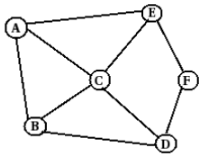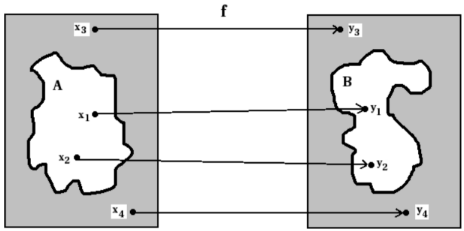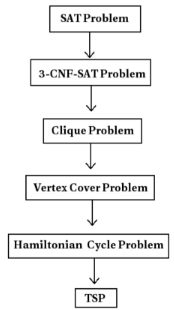# Complexity, Complexity Class P and Complexity Class NP Computer Science Engineering (CSE) Notes | EduRev

## Computer Science Engineering (CSE) : Complexity, Complexity Class P and Complexity Class NP Computer Science Engineering (CSE) Notes | EduRev

The document Complexity, Complexity Class P and Complexity Class NP Computer Science Engineering (CSE) Notes | EduRev is a part of the Computer Science Engineering (CSE) Course Theory of Computation.
All you need of Computer Science Engineering (CSE) at this link: Computer Science Engineering (CSE)

Part I. Complexity
In this module, we will learn different complexity classes, such as P and NP.
In data structures, we already learned about time complexity and space complexity. Here we will be concerned about time complexity of various problems.
We learned computable and non computable functions.
A computable function is a function for which we can construct a Turing machine. Or an algorithm can be formulated.
A non computable function is one in which no Turing machine can be constructed. Here we cannot devise an algorithm.

1 Tractable and Intractable Problems
Consider computable functions. These functions are computable or solvable. We can construct a TM for them. We can devise an algorithm for them.
But many of these problems can be solved only in principle, not in practice. This is because some of the computable functions may take 1000s of years to find a solution using a computer system. Such problems are termed intractable problems. They come under complexity class NP.
Most of the problems we are familiar with can be solved within a reasonable amount of time. Such problems are said to be tractable. They come under complexity class P.

Part II. Complexity Class P
Consider some of the problems we learned in data structures, such as bubble sort, quick sort, merge sort, binary search, matrix multiplication etc..
Also we learrned that,
The time complexity of bubble sort is Θ(n2).
The time complexity of quick sort is Θ(n log n).
The time complexity of merge sort is Θ(n log n).
The time complexity of heap sort is Θ(n log n).
The time complexity of binary search is Θ(log n).
The time complexity of matrix multiplication algorithm is Θ(n3).

Consider time complexity values of all these algorithms. They are of the order of n2, n log n, log n, n3 etc.. If we calculateexact value, we get polynomials such as 5n2 + 2n + 4, nlo n + 5n + 9, 7n3 + 3n2 + 9n + 3 etc.. They are polynomials. This means that above algorithms are polynomial time algorithms. These polynomial time algorithms can be solved within a reasonable amount of time. This means these problems canbe solved in practice.
Consider the problem, sorting using bubble sort. The time complexity of bubble sort is Θ(n2).
This means if there are 10 numbers in the list, a machine will take Θ(102) time to sort. If there are 100 numbers in thelist, a machine will take Θ(1002) time to sort. If there are 1000 numbers in the list, a machine will take Θ(10002) time to sort. All these time values are reasonable. A computing machine can solve this sorting problem within a small amount of time. Almost all the algorithms we learned have time complexity values n3, n2, n log n, n, log n, etc.. That is, for most of these algorithms, the exponent of n is at most 3.
These problems can be solved within a reasonable amount of time by a computing machine or a TM. These problems come in complexity class P. But if an algorithm for a problem has time complexity Θ(n1000), it is not a reasonable amount of time. This is also a polynomial time. But for such a problem, it has observed that somebody will invent a new algorithm that has time complexity of the order of a small value of exponent such as n4 or n3.

2 Complexity Class P
The class P consists of those problems that are solvable in polynomial time.
This means these problems can be solved in time Θ(nk), where
n is the size of the input,
k is a constant.

Another defintion is,
A problem is in class P, if there exists a deterministic Turing Machine of polynomial time complexity.
Examples for problems in class P are sorting using bubble sort, quick sort, heap sort etc.. ; searching using binary search, sequential search, ... ; matrix multiplication algorithm etc.. Most of the problems we are familiar with come under class P.

P - Hard Problems
A problem A, is said to be P-hard if,
every P problem can be reduced to A.

P - Complete Problems
A problem A, is said to be P-complete if,
A is P, and
A is P-hard.

Examples for P-complete problems :
Emptiness problem for context free grammars.
Circuit Value Problem (CVP) - Given a circuit, the inputs to the circuit, and one gate in the circuit, calculate the output of that gate Linear programming - Maximize a linear function subject to linear inequality constraints Lexicographically First Depth First Search Ordering - Given a graph with fixed ordered adjacency lists, and nodes u and v, is vertex u visited before vertex v in a depth-first search induced by the order of the adjacency lists? Context Free Grammar Membership - Given a context-free grammar and a string, can that string be generated by that grammar? Horn-satisfiability: given a set of Horn clauses, is there a variable assignment which satisfies them? This is P’s version of the boolean satisfiability problem. LZW Data Compression - given strings s and t, will compressing s with an LZ78 method add t to the dictionary? (Note that for LZ77 compression such as gzip, this is much easier, as the problem reduces to "Is t in s?".)

3 Emptiness of CFG

Emptiness Problem for Context Free Grammars
The emptiness problem is whether the grammar generates any terminal strings at all.

Emptiness Problem for CFGs is P - Complete
Theorem
The emptiness problem for context-free grammars is P-complete.
Proof
Consider any context-free grammar G ={V,∑, P, S}. The emptiness of L(G) can be determined by the following algorithm.

Step 1:
Prove that the problem is P.
Mark each of the terminal symbols in ∑.
Search P for a production A → α, in which α consists only of marked symbols and A is unmarked. If such a production rule A → α exists, then mark A and repeat the process.
If the start symbol S is unmarked, then declare L(G) to be empty. Otherwise, declare L(G) to be nonempty.
The number of iterations of Step 2 is bounded above by the number of nonterminal symbols in N. Consequently, the algorithm requires polynomial time and the problem is in P.

Step 2:
Prove that the problem is P-hard
To show that the emptiness problem for context-free grammars is P-hard, consider any problem K in P.
[This part of the proof is beyond the scope of this class.]

Part III. Complexity Class NP
Complexity class NP consists of following types of problems:

NP problems,
NP-hard problems,
NP-complete problems.
Consider computable functions. For these functions, a TM exists or they are solvable. But some of the computable functions can be solved only in principle, not in practice. This is because a computing machine may take 1000s of years to solve such problems. For these problems, a polynomial time algorithm has not yet been invented. We may wish somebody will invent a polynomial time algorithm for these problems in the future. For these problems, time complexity is found to be Θ(2n). This is not polynomial time, it is superpolynomial time complexity.
When the value of n is 10, time complexity value of such a problem will be Θ(210), which is a manageable number.
When the value of n is 100, time complexity value of such a problem will be Θ(2100). This value is greater than the
number of molecules in the universe. This means, such problems cannot be solved by a a computer in practice (when n is large). They are solvable in principle only. These problems come under complexity class NP. Some examples for such problems in NP are,
Circuit satisfiability problem,
Boolean Formula satisfiability problem (SAT),
3-CNF satisfiability problem,
Clique problem,
Vertex cover problem,
Hamiltonian cycle problem,
Traveling salesman problem (TSP).

4 Complexity Class NP
The class NP consists of those problems that are “verifiable” using a polynomial time algorithm.

What do you mean by “verifiable”.
This means if somebody gives a ’certificate’ of solution for such a problem, then we can verify that the certifcate is correct in polynomial time. For example, consider the hamiltonian cycle problem,Let somebody gives us a certificate that a hamiltonian cycle, A-C-B-D-F-E-A exists in the above graph, it can be verified very easily in polynomial time. But if we are asked to find a hamiltonial cycle from the above graph, it cannot be solved in polynomial time.
Another definition is,
A problem is in class NP if there exists a non- deterministic Turing machine of polynomial time complexity.
All problems in P are also in NP. This is because all problems in P are verifiable in polynomial time. That is, P ⊆ NP.

Polynomial Time Reducibility
In some cases, a problem can be reduced to another problem.
Consider the problem of solving the linear equation, bx + c = 0.
We may transform this to the quadratic equation 0x2 + bx + c = 0. Solution of this quadratic equation is same as the solution of the given linear equation.

A problem A is reducible to another problem B, if it is possible to convert every instance of A to a corrsponding instance of B. If this reduction is possible in polynomial time, then we say that A is polynomial time reducible to B.
This is denoted as,
A ≤p B.
This means A can be reduced to B in polynomial time.

If the algorithm used for reduction is f, then if x ∈ A, iff f(x) ∈ B, and
if x ∉ A, iff f(x) ∉ B.

This is shown below:From the above figure, x1 ∈ A,
f(x1) = y1 ,
y1 ∈ B.
Also,                        x3 ∉ A,
f(x3) = y3 ,
y3 ∉ B.

5 NP - Hard Problems

A problem A, is said to be NP-hard if, every NP problem can be reduced to A in polynomial time.

Let A be a problem. We say that A is NP-hard, if
L ≤p A , for every L ∈ NP.

6 NP - Complete Problems
A problem A, is said to be NP-complete if,
A is NP, and
A is NP-hard.

Thus if we want to prove that a problem is NP complete, we need to prove that it is NP, and all NP problems can be reduced to this problem (NP-hard).

Examples for NP complete problems are,
Circuit satisfiability problem,
Boolean Formula satisfiability problem (SAT),
3-CNF satisfiability problem (3-CNF-SAT),
Clique problem,
Vertex cover problem,
Hamiltonian cycle problem,
Traveling salesman problem (TSP).

Proving that a problem is NP complete
We will prove that above problems are NP complete. The approach we use is as follows:
We will prove that Boolean Formula satisfiability problem (SAT) is NP complete.
This is done by proving that SAT is NP, and
All problems in NP are reduced to SAT.

To prove that 3-CNF-SAT is NP complete,
Prove that 3-CNF-SAT is NP.
SAT is reduced to 3-CNF-SAT. [All NP problems can be reduced to SAT. SAT can be reduced to 3-CNF-SAT. This means all NP problems can be reduced to 3-CNF-SAT.] This means 3-CNF-SAT is NP hard.

To prove that Clique problem is NP complete,
Prove that Clicque problem is NP.

3-CNF-SAT is reduced to Clique problem. [All NP problems can be reduced to SAT. SAT can be reduced to 3-CNF-SAT. 3-CNF-SAT can be reduced to Clique problem. This means all NP problems can be reduced to Clique problem.] This means Clique problem is NP hard.

To prove that vertex cover problem is NP complete,
Prove that vertex cover problem is NP.
Clique problem is reduced to vertex cover problem. [All NP problems can be reduced to SAT. SAT can be reduced to 3-CNF-SAT. 3-CNF-SAT can be reduced to Clique problem. Clique problem can be reduced to vertex cover problem. This means all NP problems can be reduced to vertex cover problem.] This means vertex cover problem is NP hard.

To prove that Hamiltonian cycle problem is NP complete,
Prove that Hamiltonian cycle problem is NP.
Vertex cover problem is reduced to Hamiltonian cycle problem. [All NP problems can be reduced to SAT. SAT can be reduced to 3-CNF-SAT. 3-CNF-SAT can be reduced to Clique problem. Clique problem can be reduced to vertex cover problem. Vertex cover problem can be reduced to Hamiltonian cycle problem. This means all NP problems can be reduced
to Hamiltonian cycle problem.] This means Hamiltonian cycle problem is NP hard.
To prove that Traveling Salesman(TSP) problem is NP complete,

Prove that TSP is NP.
Hamiltonian cycle problem is reduced to TSP. [All NP problems can be reduced to SAT. SAT can be reducedto 3-CNF-SAT. 3-CNF-SAT can be reduced to Clique problem. Clique problem can be reduced to vertex cover problem. Vertex cover problem can be reduced to Hamiltonian cycle problem. Hamiltonian cycle problem can be reduced to TSP.
This means all NP problems can be reduced to TSP.] This means TSP is NP hard.
Thus approach we use for proving a problem is NP-complete is shown below.Offer running on EduRev: Apply code STAYHOME200 to get INR 200 off on our premium plan EduRev Infinity!

## Theory of Computation

36 videos|39 docs|39 tests

,

,

,

,

,

,

,

,

,

,

,

,

,

,

,

,

,

,

,

,

,

,

,

,

;